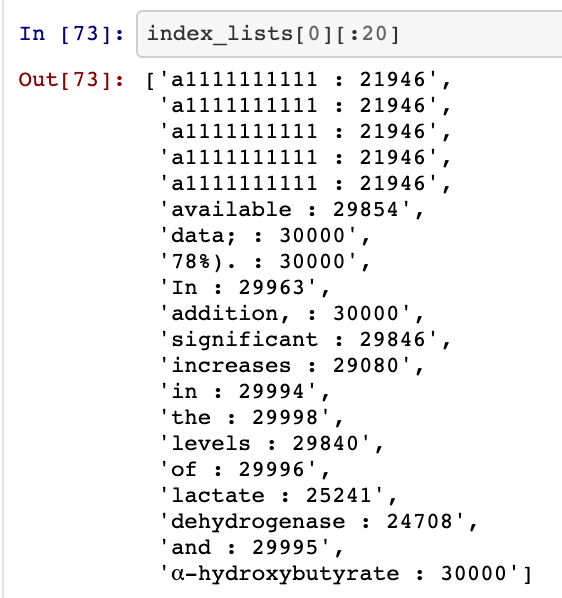# Tokenizing CORD-19 with NLTKExample of tokens mapped to their index positions. Clearly we have problems from the NLTK tokenization such as the “data;” token. I will build on this notebook with more data cleaning in the future to avoid these problems. For now, I think this is a solid tutorial on the basics of building token to index lists.

# NLTK Tokenizer

`import nltknltk.download('punkt');from nltk.tokenize import word_tokenizetext = "Exploring the CORD19 Dataset"print(word_tokenize(text))# ['Exploring', 'the', 'CORD19', 'Dataset']`
`%%timetext = "Exploring the CORD19 Dataset"word_tokenize(text);# CPU times: user 279 µs, sys: 0ns, total: 279 µs# Wall time: 293 µs`
`%timeword_tokenize(longer_text);# CPU times: user 11.8 ms, sys: 0ns, total: 11.8ms# Wall time: 11.7 ms`

# Top-K Dictionary

`%%timefreq_counter = {} # freq short for frequency...for i in df.Sequence: # Sequence is a DataFrame column containing paragraphs     tok_seq = word_tokenize(i)  for tok in tok_seq:    if tok in freq_counter.keys():      freq_counter[tok] += 1    else:      freq_counter[tok] = 1# CPU times: user 5min 5s, sys: 770 ms, total: 5min 6s# Wall time: 5min 7s`
`import operatorsorted_dict = sorted(freq_counter.items(), key=operator.itemgetter(1))sorted_dict[-2:]# [('the', 1567251), (',', 1867586)]`
`print(len(sorted_dict))print(sorted_dict[-30_000:][:5]# 656159# [('scintillation', 41),   ('inositol', 41),   ('Proteintech', 41),   ('stillbirth', 41),   ('colder', 41)]`
`top_K_list = sorted_dict[-29_999:] # 29999 -> 29_999... much more readable`
`top_K_token_to_index_dict = {}top_K_index_to_token_dict = {} # ^ verbose naming, but hopefully more clear for sake of tutorialfor i in range(len(top_K_list)):  top_K_token_index_dict[top_K_list[i]] = counter  top_K_index_token_dict[counter] = top_K_list[i]  counter += 1top_K_index_token_dict[30_000] = "Unknown"`
`import json# Token -> Indextoken_index_dict_write = json.dumps(top_K_token_index_dict)f = open("token_index_dict.json", "w")f.write(token_index_dict_write)f.close()# Index -> Tokenindex_token_dict_write = json.dumps(top_K_index_token_dict)f = open("index_token_dict.json", "w")f.write(index_token_dict_write)f.close()`
`f = open("token_index_dict.json", "r")dict_text = f.readlines()token_index_dict = json.loads(dict_text)`

# Text -> Index Mapping

`def text_to_index(seq, token_index_dict):  idx_lst = []  tok_lst = word_tokenize(seq)  for tok in tok_lst:    if tok not in token_index_dict.keys():      idx_lst.append(30_000)    else:      idx_lst.append(token_index_dict[tok])  return idx_lst`
`sentence = "hello how are you doing"text_to_index(sentence, top_K_token_index_dict)# [30_000, 29675, 29978, 29143, 25834]`

# Build Index Lists

`def build_index_lists(df, text_col_name, text_index_dict):  index_lists = []  for seq in df[text_col_name]:    seq = seq.split(' ')    new_index_list = []    for tok in seq:      if tok in text_index_dict.keys():        new_index_list.append(text_index_dict[tok])      else:        new_index_list.append(30_000)    index_lists.append(new_index_list)  return index_lists`

# Padding or Truncating Sequences to Length k (k=128 in this case)

`def pad_to_length_k(org_index_lists, k):  index_lists = org_index_lists  for seq_list = index_lists:    while (len(seq_list) > k):      seq_list.pop()    while (len(seq_list) < k):      seq_list.append(0)  return index_lists`
`index_lists = pad_to_length_k(index_lists, 128)df["Index_Lists"] = index_listsdf.info()`
`df.to_csv('IdxLists_Pdf_Json_1.csv', index=False)`

# Tokenization Complete: What we need for Downstream Applications

`from google.colab import filesfiles.upload()`
`df = pd.read_csv('IdxLists_Pdf_Json_1.csv')`
`f = open("token_index_dict.json", "r")dict_text = f.readlines()token_index_dict = json.loads(dict_text)`
`f = open("index_token_dict.json", "r")dict_text = f.readlines()index_token_dict = json.loads(dict_text)`
`git clone https://github.com/CShorten/CORD-19-Mining.git`
`import sys, ossys.path.append(os.getcwd() + '/CORD-19-Mining/')# note, I'll probably group this in a /utils folder soon# so also try    " + '/CORD-19-Mining/utils/') "   if it doesn't workfrom tokenization import text_to_index, index_to_text`
`text_to_index("hello how are you", token_index_dict)`

--

--